# Labeling Figures

Label lines: To see the equation of the line when cursor reaches the graph, use Tooltip command: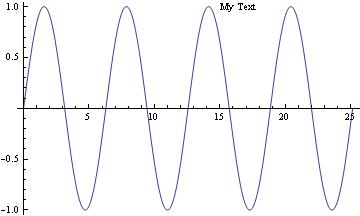Plot with title. Plot[Tooltip[Sin[x]], {x, 0, 8 Pi}] To put text/title on the picture, use Epilog command: Plot[Sin[x], {x, 0, 8 Pi}, Epilog -> Text["My Text", Offset[{32, 0}, {14, Sin}]]]

You can put title below the graph.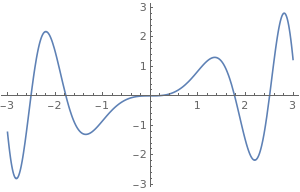The title below (visible in notebook). Clear[x]; Labeled[Plot[Sin[x^2] x, {x, -3, 3}, ImageSize -> 300], Text@TraditionalForm@Style[Sin[x*x], 16]]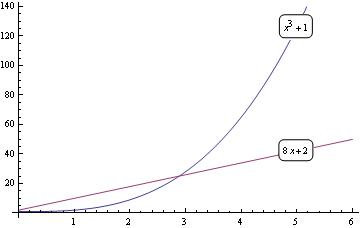Labels are included in the graph. To write labels on the graph:     fns[x_] := {1 + x^3, 2 + 8*x}; len := Length[fns[x]]; Plot[Evaluate[fns[x]], {x, 0, 6}, Epilog -> Table[Inset[ Framed[DisplayForm[fns[x][[i]]], RoundingRadius -> 5], {5, fns[[i]]}, Background -> White], {i, len}]]

You can do something similar with Locators that allows you to move the labels wherever you want: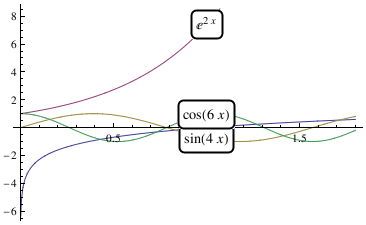Figures with locators fns[x_] := {Log[x], Exp[2*x], Sin[4*x], Cos[6*x]}; DynamicModule[{pos = Table[{1, fns[[i]]}, {i, len}]}, LocatorPane[Dynamic[pos], Plot[Evaluate[fns[x]], {x, 0, 1.8}], Appearance -> Table[Framed[Text@TraditionalForm[fns[x][[i]]], RoundingRadius -> 5, Background -> White], {i, len}]]] Export["label2.png",%]

You can use PlotLagends (that are not visible on the graph, but in Mathematica notebok):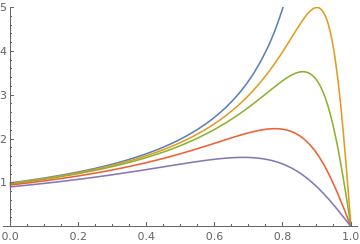Figure with legends f[a_, x_] := 1/((1 - x) (1 + a/(1 - x)^2)); parameters = {0, 0.01, 0.02, 0.05, 0.1}; Plot[Evaluate[f[#, x] & /@ parameters], {x, 0, 1}, PlotRange -> {0, 5}, PlotLegends -> Table[Row[{"a=", j}], {j, parameters}]]

Instead of PlotLagends, you can use PlotLabels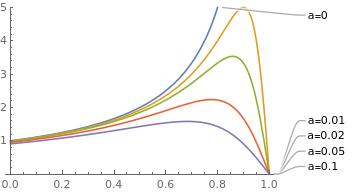Figure with labels f[a_, x_] := 1/((1 - x) (1 + a/(1 - x)^2)); parameters = {0, 0.01, 0.02, 0.05, 0.1}; Plot[Evaluate[f[#, x] & /@ parameters], {x, 0, 1}, PlotRange -> {0, 5}, PlotLabels -> Table[Row[{"a=", j}], {j, parameters}]]

Plotting with axes and without axes

There are times when the axes could interfere with displaying certain functions and solutions to ODEs. Fortunately, getting rid of axes in recent versions of Mathematica is very easy.
One method of specifying axes is to use the above options, but there is also a visual method of changing axes. Let us plot the function $$f(x) = 2\,\sin 3x -2\,\cos x$$ without ordinate but using green color and font size 12 for abscissa: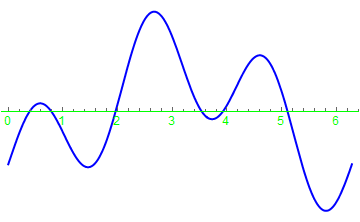Figure with abscissa only f[x_] = 2*Sin[3*x] - 2*Cos[x] Plot[f[x], {x, 0, 2*Pi}, PlotStyle -> {Thick, Blue}, Axes -> {True, False}, AxesStyle -> Directive[Green, 12]]

One can also use different colors and fonts for different axes.Figure with two axes Plot[f[x], {x, 0, 2*Pi}, PlotStyle -> {Thick, Blue}, Axes -> {True}, AxesStyle -> {Directive[Green, 12], Red}]

We can plot two graphs in the same figure:

Plot[{-1, 1} Sqrt[1 - x^2], {x, -1, 1}, PlotStyle -> {Thickness[0.007], Black}, AspectRatio -> Automatic, PlotLabel -> "Circle", LabelStyle -> Directive[Orange, Italic]]
You can also label the graph with Epilog option:
Plot[{-1, 1} Sqrt[1 - x^2], {x, -1, 1}, PlotStyle -> {Thickness[0.007], Black}, AspectRatio -> Automatic, Epilog -> Text[Style["Circle", FontSize -> 16], {0.6, 0.4}]]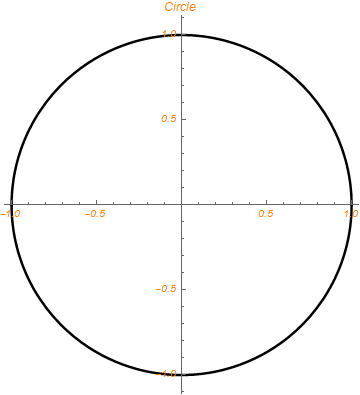We can also add title in different styles:
Plot[f[x], {x, 0, 2*Pi}, PlotStyle -> {Thick, Blue}, Axes -> {True},
AxesStyle -> {Directive[Green, 12], Red}, PlotLabel -> 2*Sin[3*x] - 2*Cos[x]] Plot[f[x], {x, 0, 2*Pi}, PlotStyle -> {Thick, Blue}, Axes -> {True},
AxesStyle -> {Directive[Green, 12], Red},
PlotLabel -> Style[Framed[2*Sin[3*x] - 2*Cos[x]], 16, Black, Background -> Lighter[Yellow]]]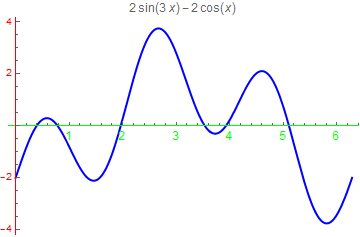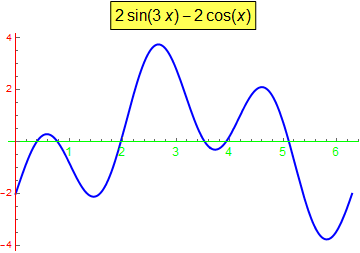We show how to present data using GraphicsGrid command:
GraphicsGrid[ Partition[ Table[Show[CountryData[c, "Flag"], PlotLabel -> c], {c, Take[CountryData["Europe"], 16]}], 4]]